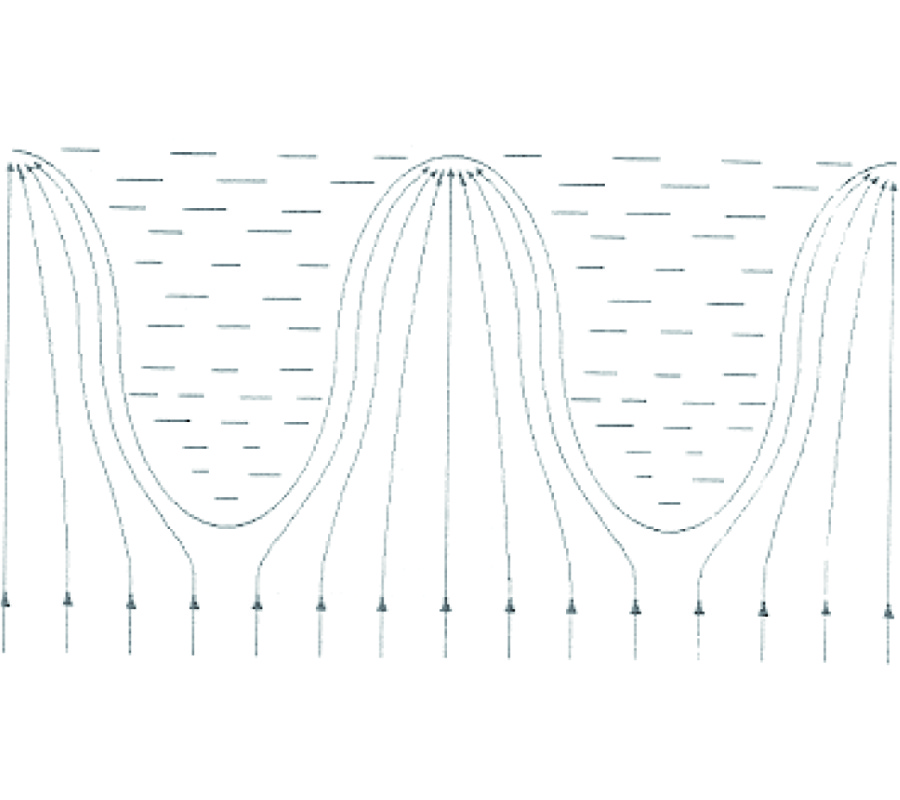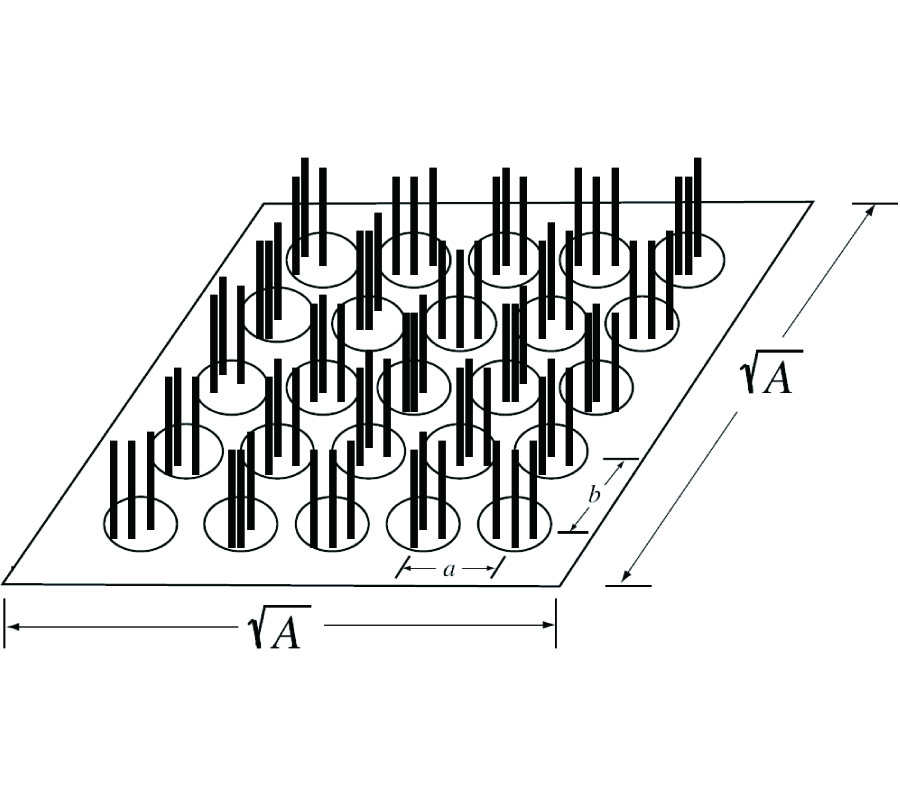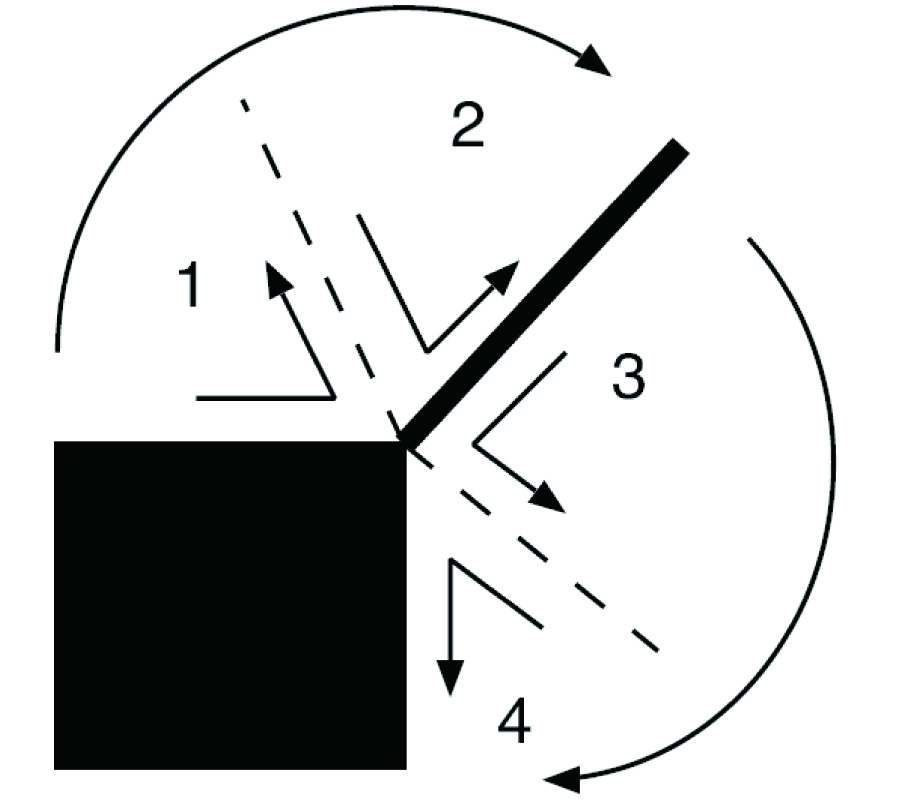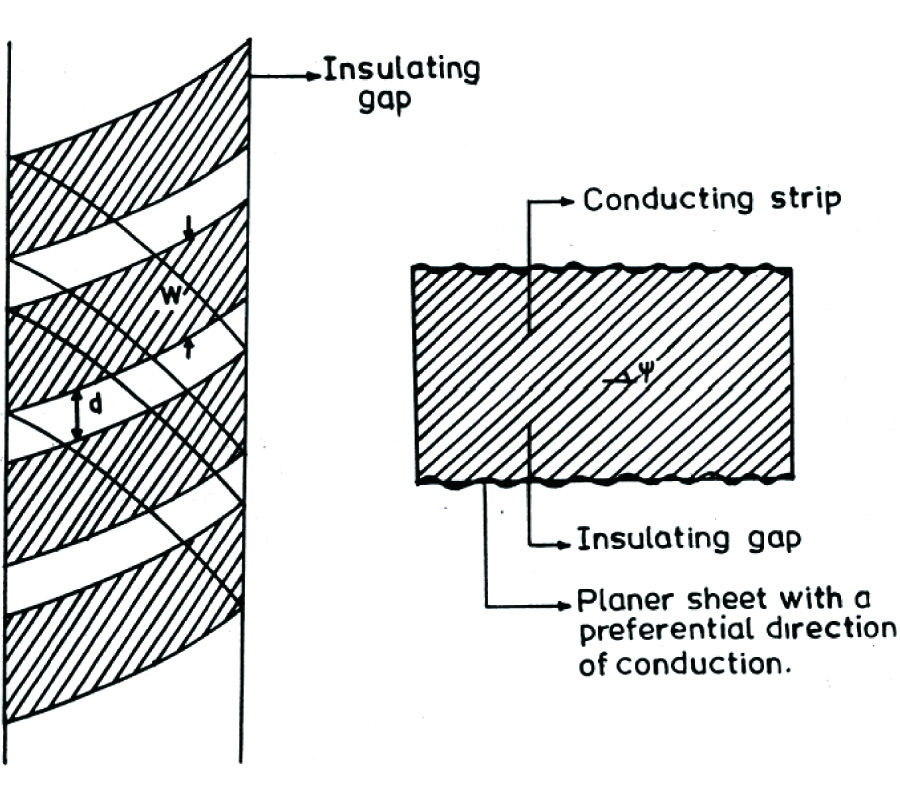ISSN: 1070-4698

# Vol. 52

Latest Volume
All Volumes
All Issues

2004-12-13 PIER Vol. 52, 321-333, 2005. doi:10.2528/PIER04100101

###Electromagnetic Scattering from Periodic Arrays of Composite Circular Cylindrer with Internal Cylindrical Scatterers

Hiroshi Toyama and Kiyotoshi Yasumoto

A very efficient and accurate method to characterize the electromagnetic scattering from periodic arrays of two-dimensional composite cylindrical ob jects with internal eccentric cylindrical scatterers is presented, using the lattice sums formula and the aggregate T-matrix for cylindrical structures. The method is quite general and applies to various configurations of two-dimensional periodic arrays. The dielectric host cylinder per unit cell of the array can contain two or more eccentric cylindrical scatterers (we call them inclusions in this paper), which may be dielectric, conductor, gyrotropic medium, or their mixture with different sizes. The power reflection coefficients from one-layer or one-hundred-layered periodic arrays of composite cylinders with up to two inclusions have been numerically studied. The effect of the presence of inclusions on the properties of resonance peaks or the stopband's width will be discussed.

2004-12-13 PIER Vol. 52, 301-320, 2005. doi:10.2528/PIER04092001

### Relativistic Electrodynamics: Various Postulate and Ratiocination Frameworks

Dan Censor

Presently various models consistent with Einstein's Special Relativity theory are explored. Some of these models have been introduced previously, but additional models are possible, as shown here. The topsy-turvy model changes the order of postulates and conclusions of Einstein's original theory. Another model is given in the spectral domain, with the relativistic Doppler Effect formulas replacing the Lorentz transformation. In this model a new principle tantamount to the constancy of the speed of light in vacuum is stated and analyzed, dubbed as the constancy of light slowness in vacuum. Because the slowness is derived in the spectral domain from the Doppler Effect formulas, this result is not trivially semantic. It is shown that potentials and equations of continuity can replace the Maxwell Equations used by Einstein for his "Principle of Relativity" in electrodynamics. It is also shown that defining convection currents and assuming the current-charge densities transformations can replace the Lorentz transformation. The list of feasible models representative rather than exhaustive, since parts of the models presented here can be combined to yield additional models. The two underlying elements of Einstein's original Special Relativity theory are always present: (1) the theory requires a kinematical element (e.g., the constancy of the speed of light in vacuum in Einstein's original model), and (2), a dynamical element (e.g., the form-invariance of the Maxwell Equations in all inertial systems of reference in Einsteins original model).

2004-12-13 PIER Vol. 52, 277-299, 2005. doi:10.2528/PIER04082801

###Possible Electromagnetic Nature of the Saturn's Rings: Superconductivity and Magnetic Levitation

Hypothesis of possible superconductivity of the iced matter of the rings of Saturn (based on the data of Voyager and Pioneer space missions) allow us to explain many phenomena which have not been adequately understood earlier. By introducing into planetary physics the force of magnetic levitation of the superconducting iced particle of the rings, which interact with the magnetosphere of the planet, it becomes possible to explain the origin, evolution, and dynamics of the rings; to show how the consequent precipitation of the rings' matter upon the planet was concluded; how the rings began their rotation; how they were compressed by the magnetic field into the thin disc, and how this disc was fractured into hundreds of thousands of separated rings; why in the ring B do exist "spokes"; why magnetic field lines have distortion near by ring F; why there is a variable azimuth brightness of the ring A; why the rings reflected radio waves so efficiently; why there exists strong electromagnetic radiation of the rings in the 20,4 kHz-40,2 MHz range and Saturnian kilometric radiation; why there is anomalous reflection of circularly polarized microwaves; why there are spectral anomalies of the thermal radiation of the rings; why the matter of the various rings does not mix but preserves its small-scale color differences; why there is an atmosphere of unknown origin nearby the rings of Saturn; why there are waves of density and bending waves within Saturn's rings; why planetary rings in the solar system appear only after the Belt of Asteroids (and may be the Belt of Asteroids itself is a ring for the Sun); why our planet Earth has no rings of its own.

2004-12-13 PIER Vol. 52, 255-276, 2005. doi:10.2528/PIER04082701

###Study of Generalized Resonance in Multi-Antenna System and Generalized Foster Reactance Theorem

Long Li, Hai-Xia Liu, Yan Shi, and Chang-Hong Liang

This paper begins with a complete description of the complex Poynting theorem, followed by a rigorous study of the generalized resonance in a multi-antenna system. The condition generating the generalized resonance is discussed, which is the balance of the electromagnetic fields energy stored in the antennas open system. The matrix expression of the generalized resonant factor (GRF) is derived. On this basis, the generalized Foster reactance theorem for an arbitrary antenna system is presented and radiation Q is used to further describe the generalized resonance behaviors. Some practical examples have shown that the generalized resonance may take on the phenomena of strong and sharp fields in the near zone and super-directivity in the far zone of the antenna system.

2004-12-13 PIER Vol. 52, 225-254, 2005. doi:10.2528/PIER04081801

###Antenna Modeling by Infinitesimal Dipoles Using Genetic Algorithms

Taninder Sijher and Ahmed Kishk

The binary Genetic Algorithm (GA) optimization method is used to simulate antennas from their near-field distribution by a set of infinitesimal dipoles. The infinitesimal dipoles could be of electric and/or magnetic types that produce the near field of the actual antenna and thus the same far field. The method is verified using near fields from known infinitesimal electric and/or magnetic dipoles. Some simple antennas have been simulated by infinitesimal dipoles such as dipole, loop, waveguide, and dielectric resonator antenna. The obtained equivalent dipoles from single frequency measurements are found to be valid for certain frequency band.

2004-12-13 PIER Vol. 52, 205-224, 2005. doi:10.2528/PIER04080903

###A Combined BI-Cgstab (1) and Wavelet Transform Method for EM Problems Using Method of Moments

An efficient technique for the solution of large-scale electromagnetic radiation and scattering problems arising from the surface integral equations and the method of moments is developed. The conventional MoM basis and testing functions are used to discretize the integral equations resulting in a dense impedance matrix. A block-partitioned wavelet transform is then employed to sparsify the matrix. Full advantage is taken of the sparse nature of the mathematical model to solve the system of equations by means of the recently introduced Stabilized Bi-Conjugate Gradient method (Bi-CGSTAB (l)). Various problems are considered involving perfect electric conductor and dielectric material. Results are compared to the corresponding results obtained via the direct solution, or LU decomposition, of the original MoM dense matrix. Excellent results are obtained in a very efficient manner. By block partitioning the MoM impedance matrix as it is built and performing the wavelet transform on the matrix blocks, analysis of very large electromagnetic problems becomes possible in a very efficient and accurate manner.

2004-12-13 PIER Vol. 52, 185-204, 2005. doi:10.2528/PIER04080902

###On the Influence of Incomplete Radiation Pattern Data on the Accuracy of a Spherical Wave Expansion

Paivi Koivisto and Johan Sten

The accuracy of a spherical wave expansion is examined when the expansion is calculated from incomplete data of the radiation pattern, i.e., when field data on a part of the far-field sphere is missing. The effect of antenna size and truncation index on the interpolation capacity of a SWE is examined by using an analytical expression for the radiation pattern of wire antennas of different lengths. The error of the SWE is seen to increase drastically when the smallest diameter of the dead zone surpasses the length of a period of the highest included wave function. The influence of the size and shape of the dead zone is studied by the aid of a measured pattern, of which a part of the field data is ignored. Two different ways are proposed for estimating the accuracy of the obtained SWE in a practical instance, when the field in the dead zone is unknown.

2004-12-13 PIER Vol. 52, 173-183, 2005. doi:10.2528/PIER04080901

###FDTD Analysis of Microstrip Patch Antenna Covered by Plasma Sheath

Z. H. Qian, Ru-Shan Chen, Kwok Leung, and Hong Yang

In this paper, a microstrip inset-fed patch antenna covered by plasma sheath is simulated by using the (FD)2TD algorithm. Expressions of calculating the coefficients in the electric field update equation for cold plasma are presented in detail. Computational examples illustrate that the resonant frequency of the patch antenna covered by plasma sheath is changed. The curves presented in this paper may be useful when introducing appropriate corrections in the design of the microstrip patch antennas in the plasma environment.

2004-12-13 PIER Vol. 52, 153-171, 2005. doi:10.2528/PIER04080601

###Electromagnetic Scattering Model for Rice Canopy Based on Monte Carlo Simulation

Li-Fang Wang, Jin Au Kong, K. Ding, T. Le Toan, F. Ribbes, and N. Floury

A scattering model for rice canopy based on Monte Carlo simulations is applied to interpret RADARSAT data and to predict the temporal response of rice growth. The model takes into account the coherent wave interactions among vegetative elements which usually occur in clusters with closely spaced elements. The model was also used to analyze the structural effect of rice fields on the scattering returns. Simulation results show a significant difference in L-band backscattering returns from rice fields with different structures, and are consistent with empirical observations from JERS-1 Campaign. Taking the ratio of HH over VV can help eliminating ambiguities in this inverse scattering problem.

2004-12-13 PIER Vol. 52, 129-151, 2005. doi:10.2528/PIER04073004

###A Low-Order-Singularity Electric-Field Integral Equation Solvable with Pulse Basis Functions and Point Matching

Robert Shore and Arthur Yaghjian

The conventional form of the electric-field integral equation (EFIE), unlike the magnetic-field integral equation, cannot be solved accurately with the method of moments using pulse basis functions and point matching. A new form of the EFIE is derived whose kernel has no greater singularity than that of the free-space Green's function. This low-order-singularity form of the EFIE, the LEFIE, is solved numerically for perfectly electrically conducting bodies of revolution (BORs) using pulse basis functions and point-matching. Derivatives of the current are approximated with finite differences using a quadratic Lagrangian interpolation polynomial. Such a simple solution of the LEFIE is contingent, however, upon the vanishing of a linear integral that appears when the original EFIE is transformed to obtain the LEFIE. This generally restricts the applicability of the LEFIE to smooth closed scatterers. Bistatic scattering calculations performed for a prolate spheroid demonstrate that results comparable in accuracy to those of the conventionally solved EFIE can be obtained with the LEFIE using pulse basis functions and point matching provided a higher density of points is used close to the ends of the BOR.

2004-12-13 PIER Vol. 52, 109-128, 2005. doi:10.2528/PIER04072101

###Error Estimation of the Method of Auxiliary Sources (Mas) for Scattering from an Impedance Circular Cylinder

Hristos Anastassiu

The purpose of this paper is a rigorous error estimation of the Method of Auxiliary Sources (MAS), when the latter is applied to electromagnetic scattering from a circular, coated, perfectly conducting cylinder, assumed to satisfy the Standard Impedance Boundary Condition (SIBC). The MAS matrix is inverted analytically, via eigenvalue analysis, and an exact expression for the discretization error in the boundary condition is derived. Furthermore, an analytical formula for the condition number of the linear system is also extracted, in addition to an asymptotic estimate for large scatterers, explaining the irregular behavior of the computational error resulting from numerical matrix inversion. Finally, the optimal location of the auxiliary sources is determined, on the grounds of error minimization.

2004-12-13 PIER Vol. 52, 81-108, 2005. doi:10.2528/PIER04071301

###Surface Integral Equation Method for General Composite Metallic and Dielectric Structures with Junctions

Pasi Yla-Oijala, Matti Taskinen, and Jukka Sarvas

The surface integral equation method is applied for the electromagnetic analysis of general metallic and dielectric structures of arbitrary shape. The method is based on the EFIE-CFIE-PMCHWT integral equation formulation with Galerkins type discretization. The numerical implementation is divided into three independent steps: First, the electric and magnetic field integral equations are presented and discretized individually in each non-metallic subdomain with the RWG basis and testing functions. Next the linearly dependent and zero unknowns are removed from the discretized system by enforcing the electromagnetic boundary conditions on interfaces and at junctions. Finally, the extra equations are removed by applying the wanted integral equation formulation, and the reduced system is solved. The division into these three steps has two advantages. Firstly, it greatly simplifies the treatment of composite ob jects with multiple metallic and dielectric regions and junctions since the boundary conditions are separated from the discretization and integral equation formulation. In particular, no special junction basis functions or special testing procedures at junctions are needed. Secondly, the separation of the integral equation formulation from the two previous steps makes it easy to modify the procedure for other formulations. The method is validated by numerical examples.

2004-12-13 PIER Vol. 52, 47-80, 2005. doi:10.2528/PIER04070201

###Analytical Techniques to Evaluate the Integrals of 3D and 2D Spatial Dyadic Green's Functions

Guozhong Gao, Carlos Torres-Verdin, and Tarek Habashy

The Dyadic Green's function is in general viewed as a generalized, or distribution function. A commonly used procedure to evaluate its volume integral is the principal-volume method, in which an infinitesimal volume around the singularity is excluded from the integration volume. In this paper, we develop a general analytical technique to evaluate the integral of the dyadic Green's function without the need to specify an exclusion volume. The newly derived expressions accurately integrate the singularity and can be used for integration over any shape of spatial discretization cell. We derive explicit expressions for the integral of the 3D dyadic Green's function over a sphere and over a general rectangular block. Similar expressions are obtained for the 2D dyadic Green's function over a cylinder and over a general rectangular cell. It is shown that using the integration technique described in this paper for spherical/circular cells, simple analytical expressions can be derived, and these expressions are exactly the same as those obtained using the principal-volume method. Furthermore, the analytical expressions for the integral of the dyadic Green's function are valid regardless of the location of the observation point, both inside and outside the integration domain. Because the expressions only involve surface integrals/line integrals, their evaluation can be performed very efficiently with a high degree of accuracy. We compare our expressions against the equivalent volume approximation for a wide range of frequencies and cell sizes. These comparisons clearly show the efficiency and accuracy of our technique. It is also shown that the cubic cell (3D) and the square cell (2D) can be approximated with an equivalent spherical cell and circular cell, respectively, over a wide range of frequencies. The approximation can be performed analytically, and the results can be written as the value of the dyadic Green's function at the center multiplying a "geometric factor". We describe analytical procedures to derive the corresponding geometric factors.

2004-12-13 PIER Vol. 52, 23-46, 2005. doi:10.2528/PIER04062501

###Analysis of the Temperature Increase Linked to the Power Induced by RF Source

Ahmed Ibrahiem, Christian Dale, Walid Tabbara, and Joe Wiart

Temperature increase analysis has been performed with consideration to the anatomical model of the human head exposed to a cellular phone operating at 900 MHz. Four different numerical methods, in particular an implicit method based on the Alternating Direction Implicit technique (ADI), were applied to solve the Bio-Heat Equation (BHE), their advantages and limitations were compared using a canonical case. The tests performed on the latest have shown that the implicit approach is well adapted to solve this type of equations. The rise of temperature in the human head exposed to the RF emission of a mobile phone with a radiated power of 250 mW at 900 MHz was analyzed. In addition the influence of the presence of the telephone kit close to the head was discussed. The influence of different thermal parameters such as the thermal conductivity and the blood perfusion coefficient on the rise of temperature has been analyzed. The simulation carried out showed that the maximum temperature increase in the internal tissues linked to SAR deposition does not exceed 0.1ºC. We recorded a temperature difference of 1.6ºC in the skin due to the presence of a switched off cellular phone, which has been confirmed by the experimental measurements performed.

2004-12-13 PIER Vol. 52, 1-21, 2005. doi:10.2528/PIER04052002

###Elliptical and Circular Step-Index Fibers with Conducting Helical Windings on the Core-Cladding Boundaries for Different Winding Pitch Angles - a Comparative Modal Dispersion Analysis

Deepak Kumar and Onkar Singh II

The propagation characteristics of an elliptical step-index fiber with a conducting helical winding on the core-cladding boundary are investigated analytically and compared with those of a circular step index fiber with a conducting radial winding. Our optical waveguides are unconventional: in view of the existence of helical conducting windings on the core-cladding boundaries. Appropriate coordinate systems, circular cylindrical and elliptic cylindrical, are chosen for the circular and elliptical fibers. Applying the boundary conditions as modified by the presence of conducting helical windings, the characteristic equations are obtained for both the fibers. Dispersion curves are also obtained for two special values of the helical pitch angle ψ, namely, for ψ = 0º and ψ = π/2 for each fiber and the results have been compared. It is found that the introduction of the helical winding has two main effects on the characteristics of both types of fibers. These are: (1) The helix introduces band gaps and (2) has the effect of splitting a mode into a pair of adjacent modes In the case of the elliptical helically clad waveguide we find two band gaps for V < 30 whereas for circular guide we have only one band gap in the same range of V-values, V being the normalized frequency parameter.